# Quick Sort

Similar to merge sort, quick sort is embedded within the sorting primitives of many modern languages1. While, strictly speaking, its asymptotic complexity is inferior to merge sort’s, it often outperforms merge sort in practice. This is largely due to the fact that quick sort is an in place sorting algorithm while merge sort copies all the data to an entirely new memory location.

#### History

Sir Charles Antony Richard Hoare discovered the quick sort algorithm in 1960 while working on language translation software2. Mr. Hoare earned many prestigious accolades throughout his career including a Turing award in 1980 and the Kyoto Prize in 2000. His most memorable, some would say infamous, accomplishment is the conception of “NULL” values which he implemented for ALGOL in 1965. Since then, the majority of modern languages have followed suit. Mr. Hoare is quoted as classifying null reference errors as his “billion-dollar mistake”.

Another attribute that merge and quick sort have in common is that they are both examples of the divide and conquer algorithm design paradigm. Recall that this basic recipe consists of:

1. Divide the input into smaller sub problems
2. Conquer the sub problems recursively
3. Combine the solutions of the sub problems into a final solution

Consulting the pseudo code, lines 5 thru 7 account for step one of the recipe. The seconds step is accomplished with lines 9 thru 11. Owing to the operation of the partition method, the third step happens automatically. The partition method is the most complex piece, so it’s prudent to provide a more in-depth explanation.

### Partition

partition accepts a list of data. The item located at index 0 becomes the partition point, also known as the pivot3. In a nut shell, it moves ordinally superior values in front of the pivot and ordinally inferior values after it4. The image below depicts a partition operation on a list of integers with a desired ascending sort order.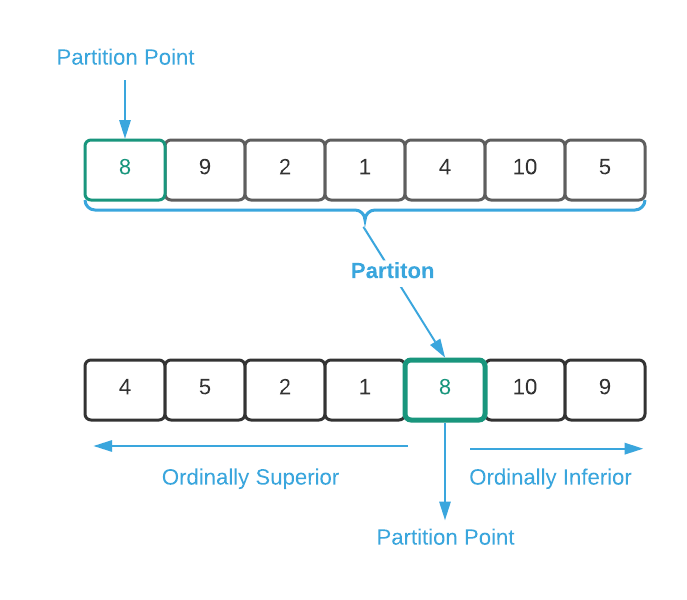The important notion here is that partition does not fully sort the data. It provides three guarantees:

1. The partition point is in it’s correct position (the same position as if the data were fully sorted)
2. All items to the left of the partition point are ordinally superior
3. All items to the right of the partition pointer are ordinally inferior

The implementation of partition is vital to the performance of the overall algorithm. Many sources employ an inefficient double loop, similar to insertion sort, due to it’s cognitive simplicity5. However, the only way to ensure that the overall asymptotic complexity of the algorithm conforms to the desired $O(n \log_2 n)$ (on average) runtime is to ensure that partition is linear ($O(n)$). Examine the pseudo code in conjunction with the animation below to understand how to properly implement partition.There is still one important detail that has been ignored to this point, that is the choosePivot method as shown in lines 40 thru 42 of the pseudo code. That is the topic of the next subsection.

### Choose Pivot

The purpose of choosePivot is to identify the value in which to partition around. Lines 5 and 6 of the pseudo code describe its use. Removing those two lines has no effect on the end state. This begs the question, why use it? The answer is that it ensures a consistent runtime regardless of the ordering of the input data. This is a bit difficult to comprehend without examples.

First, consider the ideal scenario where the pivot element is always the median value as depicted below6. The asymptotic complexity is better than $O(n \log_2 n)$ in this case. As proof, first note that $n = 7$ meaning there are 7 elements in the list of data. Plugging $n$ into the runtime yields $O(7 \log_2 7) \approx O(20)$. So, the assertion is that the total number of operations is less than 20. partition is involved 3 times: once with 7 elements and twice with 3 elements. Assuming that partition is linear, that’s a total of 13 operations which validates the assertion.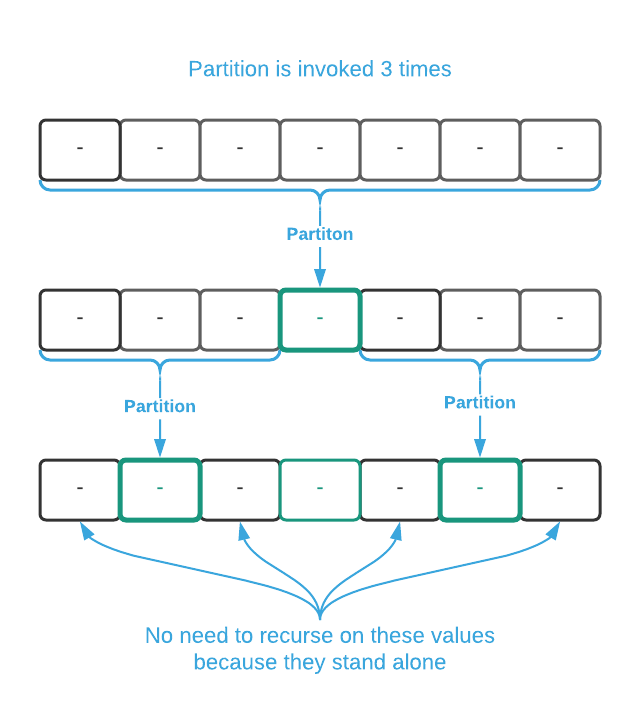Now consider the other end of the spectrum where the pivot element is always the most ordinally superior value. Using the image below as a guide, partition is involved six times with input sizes of 7, 6, 5, 4, 3, and 2 for a total of 27 operations. That’s more than twice the number of operations in the previous example.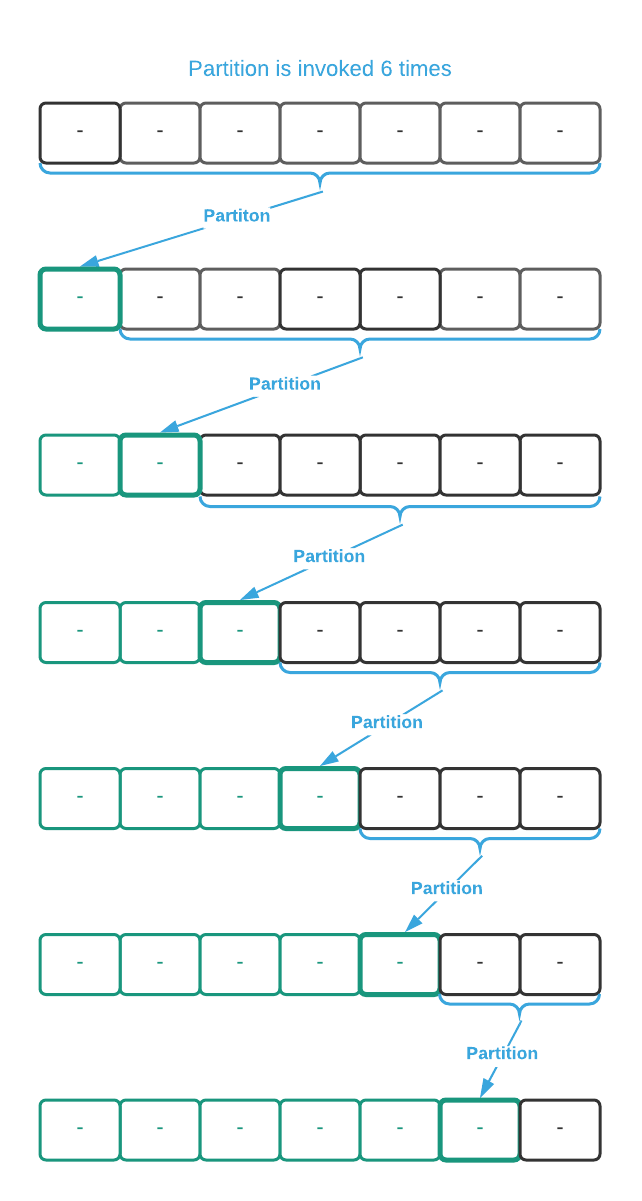The next question becomes, is it possible to divine the median value? The answer is, unfortunately, no7. Determining the median is more computationally intensive than an inefficient sort. One solution is to partition on the first value (index 0). This is equivalent to removing lines 5 and 6 from the pseudo code. This works just as well as any other strategy if the input data is randomly ordered. The problem is that pre-sorted data results in the worst case scenario. Likewise, partitioning on last value (index $n-1$) has the same effect. Choosing the pivot element at random, as shown in the pseudo code, ensures an acceptable runtime regardless of the input data. In fact, it’s proven to result in a $O(n \log_2 n)$ runtime on average8.

To make this concept a bit more concrete, the data below compares the actual runtimes9 for sorting data with the following pivot strategies.

• Pivot on Last: choosePivot returns $n-1$
• Pivot on First: choosePivot returns $0$
• Pivot on Random: choosePivot as depicted in the pseudo code

#### Randomly Ordered Input Data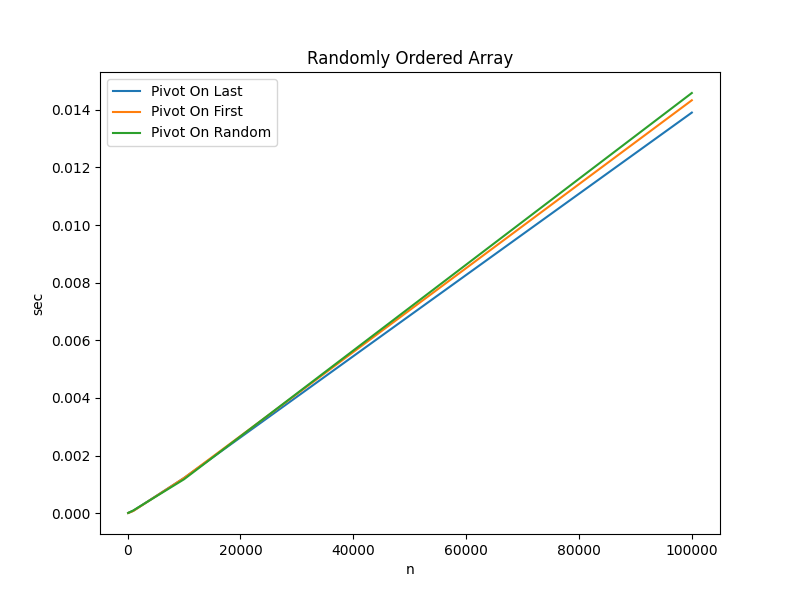Algorithm n=100 n=1000 n=10000 n=100000
Pivot On Last 0.000007 sec 0.000081 sec 0.001220 sec 0.013905 sec
Pivot On First 0.000005 sec 0.000075 sec 0.001221 sec 0.014333 sec
Pivot On Random 0.000008 sec 0.000093 sec 0.001176 sec 0.014585 sec

Key Takeaways:

• When the input data is randomly ordered, the choice of pivot is inconsequential

#### Input Data Sorted in Ascending Order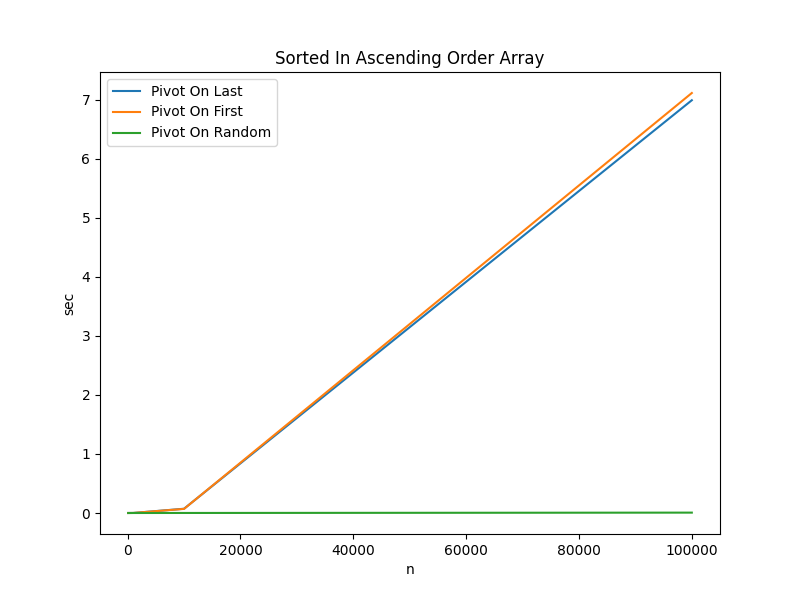Algorithm n=100 n=1000 n=10000 n=100000
Pivot On Last 0.000010 sec 0.000714 sec 0.072023 sec 6.988404 sec
Pivot On First 0.000009 sec 0.000698 sec 0.069650 sec 7.110827 sec
Pivot On Random 0.000006 sec 0.000051 sec 0.000569 sec 0.006392 sec

Key Takeaways:

• When the input data is pre-sorted, selecting the pivot randomly is close to 1,500 times faster
• The random pivot strategy is actually slightly more efficient with pre-sorted data because partitions require fewer swaps

#### Input Data Sorted in Descending Order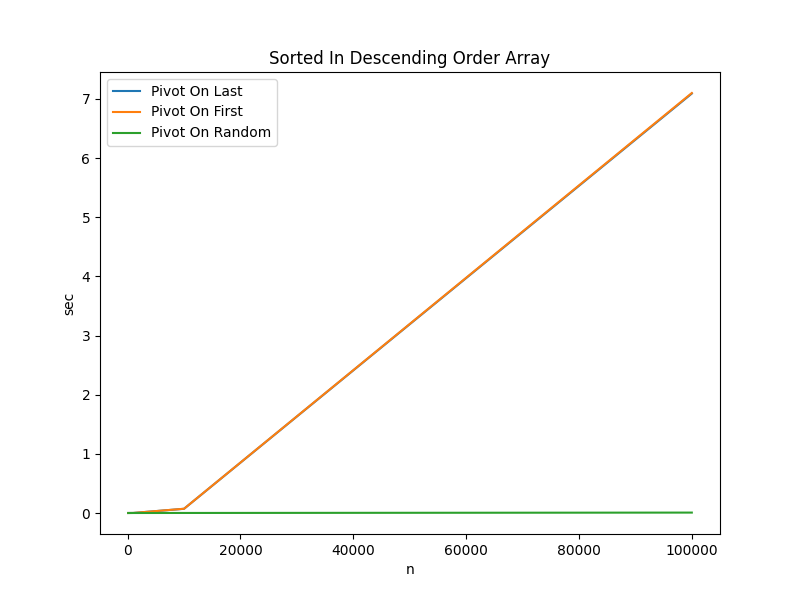Algorithm n=100 n=1000 n=10000 n=100000
Pivot On Last 0.000011 sec 0.000727 sec 0.070983 sec 7.089717 sec
Pivot On First 0.000010 sec 0.000735 sec 0.071918 sec 7.099061 sec
Pivot On Random 0.000007 sec 0.000054 sec 0.000634 sec 0.006950 sec

Key Takeaways:

• The results for data sorted in descending order are similar to the results for data sorted in ascending order

### Wrapping it Up

To bring this all together, the image below illustrates sorting a list of integers in ascending order using the quick sort algorithm. For the sake of brevity, it always pivots on the first item (index 0). Because the input data is randomly ordered, this isn’t detrimental to performance.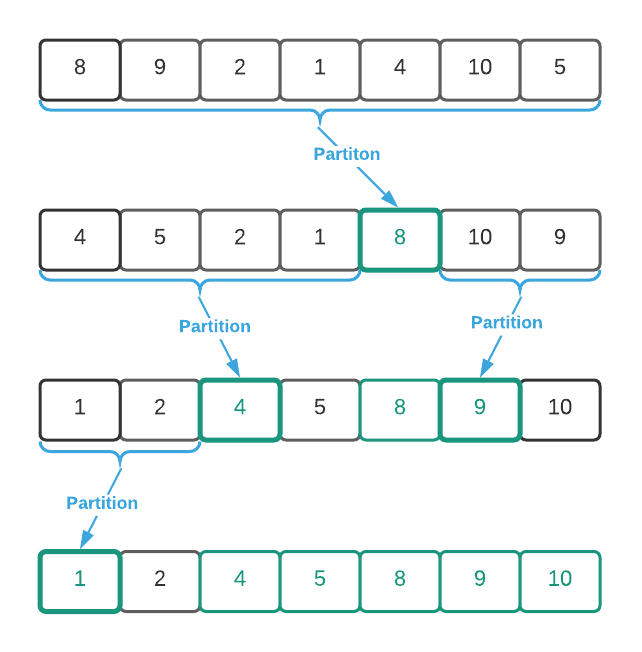## Pseudo Code

\begin{algorithm}
\caption{Quick Sort}
\begin{algorithmic}
\REQUIRE $A$ = list of numbers
\REQUIRE $n$ = number of items to consider
\ENSURE $A$ sorted in sequential order
\FUNCTION{quickSort}{A, n}
\IF{$n \leq 1$}
\RETURN
\ENDIF
\STATE pivot $\gets$ \CALL{choosePivot}{n}
\STATE swap $A_0$ and $A_{\text{pivot}}$
\STATE pivot $\gets$ \CALL{partition}{A}
\STATE
\STATE \CALL{quickSort}{$A_0...A_{\text{pivot - 1}}$, pivot}
\STATE pivot $\gets$ pivot + 1
\STATE \CALL{quickSort}{$A_{\text{pivot}}...A_{\text{n-pivot-1}}$, n - pivot}
\ENDFUNCTION
\STATE
\REQUIRE $A$ = list of numbers where $A_0$ is the value to partition around
\ENSURE All values $\lt A_0$ arranged before $A_0$ and values $\gt A_0$ arranged after $A_0$
\OUTPUT position of the value at $A_0$ after the partition
\FUNCTION{partition}{A}
\STATE low $\gets$ 1
\STATE high $\gets \vert A \vert - 1$
\STATE
\WHILE{true}
\WHILE{low $\lt$ high and $A_{\text{low}} \lt A_0$}
\STATE low $\gets$ low + 1
\ENDWHILE
\STATE
\WHILE{high $\geq$ low and $A_{\text{high}} \geq A_0$}
\STATE high $\gets$ high - 1
\ENDWHILE
\STATE
\IF{low $\geq$ high}
\BREAK
\ENDIF
\STATE
\STATE swap $A_{\text{low}}$ and $A_{\text{high}}$
\STATE low $\gets$ low + 1
\STATE high $\gets$ high - 1
\ENDWHILE
\STATE
\STATE swap $A_0$ and $A_{\text{high}}$
\RETURN{high}
\ENDFUNCTION
\STATE
\REQUIRE $n$ = number of elements in the partition
\OUTPUT ideal index to partition on
\FUNCTION{choosePivot}{n}
\RETURN uniformly random number between 0 and $n$ inclusive
\ENDFUNCTION
\end{algorithmic}
\end{algorithm}


#### Implementation Details

Notice lines 9 and 11 of the pseudo code. Essentially, what’s happening is a recursion on a portion of the input array. In many languages such as Python and ES6, it’s easy to create a slice of an array. For instance, line 9 may be written as follows:

There is a glaring problem with this approach. Creating a slice allocates a shallow copy of the array which is wasteful and has negative performance implications. A better approach is recurse on the existing array and specify the start and end index as follows:

## Asymptotic Complexity

$O(n^2)$; however, it’s $O(n \log_2 n)$ on average

Full Repo

Relevant Files:

## Exercises

1. What is the asymptotic memory consumption of the quick sort algorithm?

• $\Theta(1)$ - Quick sort is an in place sorting algorithm meaning that it sorts by swapping items within the given list. Therefore, the only additional memory required is the temporary variable used during a swap. - Strictly speaking, this answer is only partially correct; however, it’s acceptable given that this source does not cover stack memory usage with recursive algorithms.
• $O(\log_2 n)$ - Quick sort is a recursive algorithm so, assuming a non-tail call optimized language, the stack grows with each recursive call.
2. What is the result of removing lines 32 and 33 from the pseudo code considering:
a. the end state
b. runtime

1. The change has no effect on the end state of the data. It will continue to sort correctly.
2. The loop starting at line 18 will suffer two superfluous comparison operations as lines 19 and 23 will evaluate to true at least once.
3. Using the programming language of your choice, implement a quick sort function that accepts:

See the C implementation provided in the links below. Your implementation may vary significantly and still be correct.
- quick_sort.h
- quick_sort.c
- quick_sort_test.c

4. Instrument your quick sort function from the previous exercise to calculate the total number of copy and comparison operations. Next, sort the data contained in the sort.txt file and determine the total number of copy and comparison operations using a “pivot on first (index 0)” strategy.

See the C implementation provided in sort_implementation.c Your implementation may vary significantly and still be correct. The final output should be:

Quick Sort: copy = 113,769 comparisons = 171,705 total = 285,474


As a reference, here is how quick sort stacks up against the other sorting algorithms.

    Bubble Sort: copy = 71,844,390 comparisons = 49,988,559 total = 121,832,949
Insertion Sort: copy = 23,968,128 comparisons = 23,958,117 total =  47,926,245
Selection Sort: copy =     29,964 comparisons = 49,995,000 total =  50,024,964
Merge Sort: copy =    133,616 comparisons =    120,473 total =     254,089
Quick Sort: copy =    113,769 comparisons =    171,705 total =     285,474

1. To be 100% accurate, the sorting primitives provided by most languages dynamically choose algorithms depending on the input data. C++ is a good example. Additionally, introsort, an evolution of quick sort, is far more prevalent nowadays.

2. Listen to the account in Mr. Hoare’s own words here: https://www.bl.uk/voices-of-science/interviewees/tony-hoare/audio/tony-hoare-inventing-quicksort

3. Some implementations partition around the last item rather than the first. In practice, the difference is inconsequential.

4. Ordinally superior items sort before ordinally inferior items. For instance, if the desired sort order is ascending, 1 is ordinally superior to 2. When sorting in descending order, 2 is ordinally superior to 1.

5. In 99% of cases, simplicity is better. However, the goal of this algorithm is to create an optimally performant general purpose sorting utility. The trade off of cognitive complexity for performance is justified in this case.

6. Data values are excluded to keep the examples simple.

7. Determining the median value in a list of unsorted data is, at best, an $O(n)$ operation. However, it’s not uncommon to determine the median value of the first, last, and middle elements in a list. See the PivotOnMedian function in the accompanying C code for an example. https://github.com/dalealleshouse/algorithms/blob/master/src/sorting/quick_sort.c

8. See section 7.4 on page 180 of Introduction to Algorithms, 3rd Edition by Thomas H. Cormen, Charles E. Leiserson, Ronald L. Rivest, and Clifford Stein for a proof of the average runtime.

9. For information about how the data was collected, see the Actual Run Times section of the Source Code page.